Using DSolve to Find  Symbolic Solutions to Differential Equations: General Homogeneous Case

Mathematica can solve the the general homogeneous first-order linear ODE: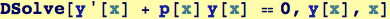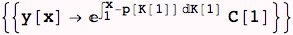The dummy integration variables  (K in the above) and any integration constants  (C above) are picked by Mathematica .  Note that we asked Mathematica to solve the most general form of homogeneous linear first-order ODE, and we got a solution in a very general form that is equivalent to that found in textbooks.  Here is a more specific problem and the solution found by Mathematica: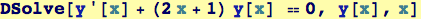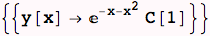There is an integration constant above, that will take on a specific value if an additional condition (such as an initial condition, or a boundary condition) is specified. Below, we stipulate that y is 4; so we should get the result with C specified; y = C = 4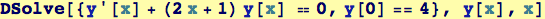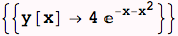Created by Wolfram Mathematica 6.0  (10 November 2007)